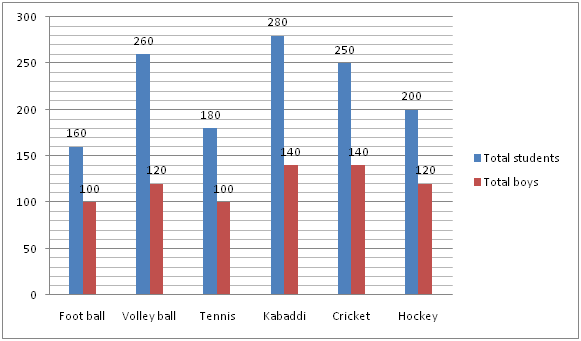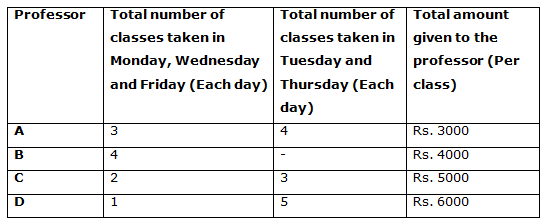# Quantitative Aptitude Questions (Data Interpretation) for Indian Bank PO Mains 2018 Day-212

Dear Readers, Indian Bank is conducting Online Examination for the recruitment of PO. To enrich your preparation here we have providing new series of Data Interpretation – Quantitative Aptitude Questions. Candidates those who are appearing in Indian Bank PO Mains Exams can practice these Quantitative Aptitude average questions daily and make your preparation effective.

[WpProQuiz 3936]

Directions (Q. 1 – 5) Study the following information carefully and answer the given questions:

The following bar graph shows the total number of students (Boys + Girls) and the number of boys participated in the different games.1) Total number of girls participated in Football is what percentage of total number of boys participated in Tennis?

a) 75 %

b) 60 %

c) 50 %

d) 40 %

e) None of these

2) Find the ratio between the total number of students participated in Volleyball and Tennis together to that of total number of girls participated in Kabaddi and Cricket together?

a) 35: 19

b) 27: 11

c) 15: 7

d) 44: 25

e) None of these

3) Find the difference between the total number of girls to that of total number of boys participated in Foot ball and Hockey together?

a) 80

b) 60

c) 50

d) 70

e) None of these

4) Find the average number of girls participated in Volley ball, Tennis and Cricket together?

a) 90

b) 120

c) 110

d) 70

e) None of these

5) Total number of students participated in Football is approximately what percentage more/less than the total number of boys participated in Kabaddi?

a) 30 % less

b) 15 % less

c) 45 % more

d) 15 % more

e) 30 % more

Directions (Q. 6 – 10) Study the following information carefully and answer the given questions:

The following table shows the number of classes taken by each professor in different days and the total amount given to the professor per class also given for the certain course.Note:

No more classes in Saturday and Sunday.

Each class taken for one hour

‘-‘ indicates the value we have to find out.

6) Find the difference between the total classes taken by the professor A in 3 weeks and that of the professor D in 4 weeks?

a) 4

b) 3

c) 1

d) 2

e) None of these

7) If the amount of Rs. 1.92 lakhs was given to the professor B for 3 weeks, then find the number of classes taken by the professor B in Thursday in 3 weeks?

a) 6

b) 9

c) 12

d) 15

e) None of these

8) Find the total amount given to the professor C in 4 weeks?

a) Rs. 218000

b) Rs. 256000

c) Rs. 272000

d) Rs. 240000

e) None of these

9) Find the difference between the total amount given to the professor A for 3 weeks and that of the total amount given to the professor D for 5 weeks?

a) Rs. 259000

b) Rs. 237000

c) Rs. 213000

d) Rs. 275000

e) None of these

10) The total amount given to the professor B per class is what percentage of the total amount given to the professor C per class?

a) 65 %

b) 105 %

c) 80 %

d) 120 %

e) 50 %

Direction (1-5) :

Explanation:

Total number of girls participated in Football = 160 – 100 = 60

Total number of boys participated in Tennis = 100

Required % = (60/100)*100 = 60 %

Explanation:

The total number of students participated in Volleyball and Tennis together

= > 260 + 180 = 440

The total number of girls participated in Kabaddi and Cricket together

= > (280 – 140) + (250 – 140)

= > 140 + 110 = 250

Required ratio = 440: 250 = 44: 25

Explanation:

The total number of girls participated in Foot ball and Hockey together

= > (160 – 100) + (200 – 120)

= > 60 + 80 = 140

The total number of boys participated in Foot ball and Hockey together

= > 100 + 120 = 220

Required difference = 220 – 140 = 80

Explanation:

The total number of girls participated in Volley ball, Tennis and Cricket together

= > (260 – 120) + (180 – 100) + (250 – 140)

= > 140 + 80 + 110 = 330

Required average = 330/3 = 110

Explanation:

Total number of students participated in Football = 160

Total number of boys participated in Kabaddi = 140

Required % = [(160 – 140)/140]*100 = 15 % more

Direction (6-10) :

Explanation:

The total classes taken by the professor A in 1 week

= > (3*3) + (4*2) = 9 + 8 = 17

The total classes taken by the professor A in 3 weeks = 17*3 = 51

The total classes taken by the professor D in 1 week

= > (1*3) + (5*2) = 3 + 10 = 13

The total classes taken by the professor D in 4 weeks = 13*4 = 52

Required difference = 52 – 51 = 1 class

Explanation:

Total number of classes taken by the professor B in 3 weeks

= > 192000/4000 = 48 classes

According to the question,

(12*3) + 3*(Tuesday + Thursday) = 48

3*(Tuesday + Thursday) = 48 – 36

3*(Tuesday + Thursday) = 12

Tuesday + Thursday = 4 classes

Total number of classes taken by professor B in Thursday = 2 classes

The number of classes taken by the professor B in Thursday in 3 weeks

= > 2*3 = 6 classes

Explanation:

The number of classes taken by the professor C in a week

= > (2*3) + (3*2) = 6 + 6 = 12

The number of classes taken by the professor C in 4 weeks

= > 12*4 = 48

The total amount given to the professor C in 4 weeks

= > 48*5000 = Rs. 240000

Explanation:

The number of classes taken by the professor A in a week

= > (3*3) + (4*2) = 9 + 8 = 17

The number of classes taken by the professor A in 3 weeks

= > 17*3 = 51

The total amount given to the professor A for 3 weeks

= > 51*3000 = Rs. 153000

The number of classes taken by the professor D in a week

= > (1*3) + (5*2) = 3 + 10 = 13

The number of classes taken by the professor D in 5 weeks

= > 13*5 = 65

The total amount given to the professor D for 5 weeks

= > 65*6000 = Rs. 390000

Required difference = 390000 – 153000 = Rs. 237000

Explanation:

The total amount given to the professor B per class = Rs. 4000

The total amount given to the professor C per class = Rs. 5000

Required % = (4000/5000)*100 = 80 %

Daily Practice Test Schedule | Good Luck

 Topic Daily Publishing Time Daily News Papers & Editorials 8.00 AM Current Affairs Quiz 9.00 AM Quantitative Aptitude “20-20” 11.00 AM Vocabulary (Based on The Hindu) 12.00 PM General Awareness “20-20” 1.00 PM English Language “20-20” 2.00 PM Reasoning Puzzles & Seating 4.00 PM Daily Current Affairs Updates 5.00 PM Data Interpretation / Application Sums (Topic Wise) 6.00 PM Reasoning Ability “20-20” 7.00 PM English Language (New Pattern Questions) 8.00 PM